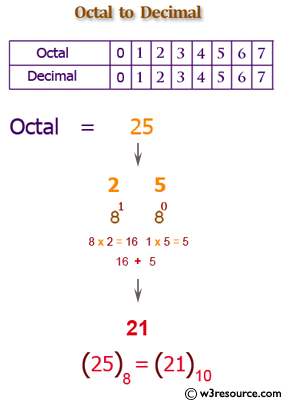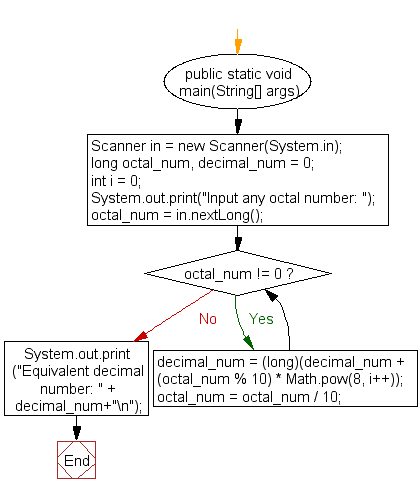﻿ Java exercises: Convert a octal number to a decimal number - w3resource# Java Exercises: Convert a octal number to a decimal number

## Java Basic: Exercise-25 with Solution

Write a Java program to convert a octal number to a decimal number.

Octal number: The octal numeral system is the base-8 number system, and uses the digits 0 to 7.

Decimal number: The decimal numeral system is the standard system for denoting integer and non-integer numbers. It is also called base-ten positional numeral system.

Test Data:
Input any octal number: 10

Pictorial Presentation: Octal to Decimal numberSample Solution:

Java Code:

``````import java.util.Scanner;

public class Exercise25 {

public static void main(String[] args)
{
Scanner in = new Scanner(System.in);
long octal_num, decimal_num = 0;
int i = 0;
System.out.print("Input any octal number: ");
octal_num = in.nextLong();
while (octal_num != 0)
{
decimal_num = (long)(decimal_num + (octal_num % 10) * Math.pow(8, i++));
octal_num = octal_num / 10;
}
System.out.print("Equivalent decimal number: " + decimal_num+"\n");
}
}
```
```

Sample Output:

```Input any octal number: 10
Equivalent decimal number: 8
```

Flowchart:Java Code Editor:

What is the difficulty level of this exercise?

Test your Programming skills with w3resource's quiz.

﻿

## Java: Tips of the Day

getEnumMap

Converts to enum to Map where key is the name and value is Enum itself.

```public static <E extends Enum<E>> Map<String, E> getEnumMap(final Class<E> enumClass) {
return Arrays.stream(enumClass.getEnumConstants())
.collect(Collectors.toMap(Enum::name, Function.identity()));
}
```

Ref: https://bit.ly/3xXcFZt